# 数据结构与算法学习之动态数组

## 什么是数据结构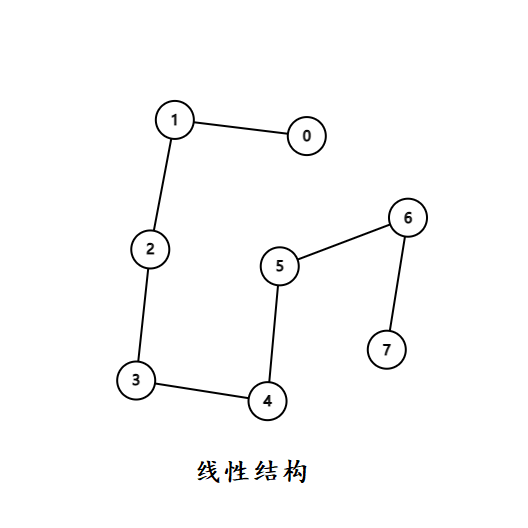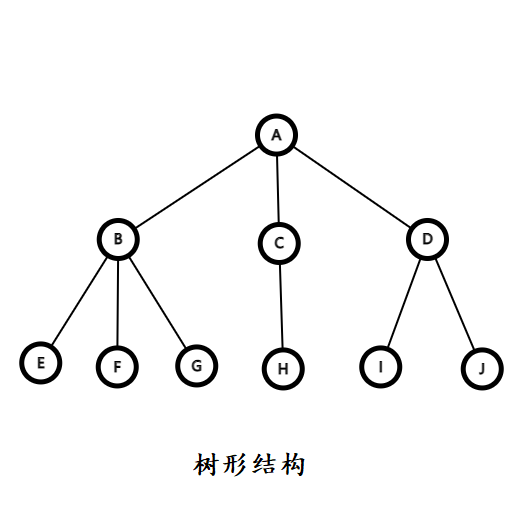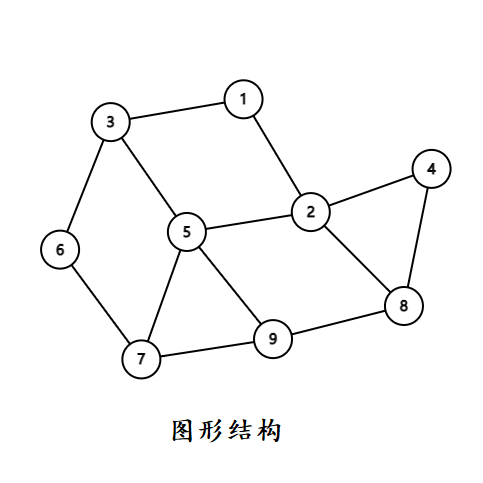## 线性表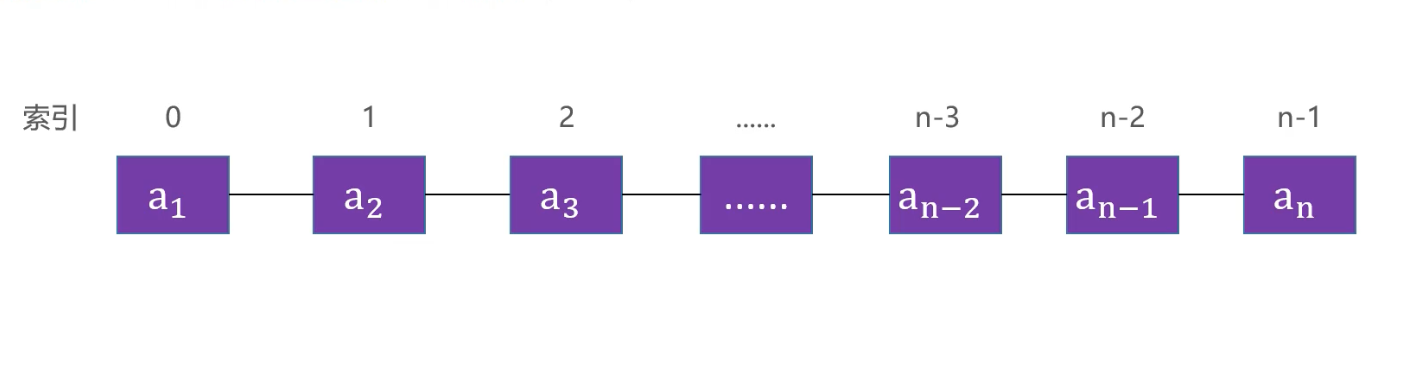• a1首节点（首元素）， an尾结点（尾元素）
• a1 是 a2前驱， a2 是 a1后继

## 数组

int[] array = new int[]{11,22,33};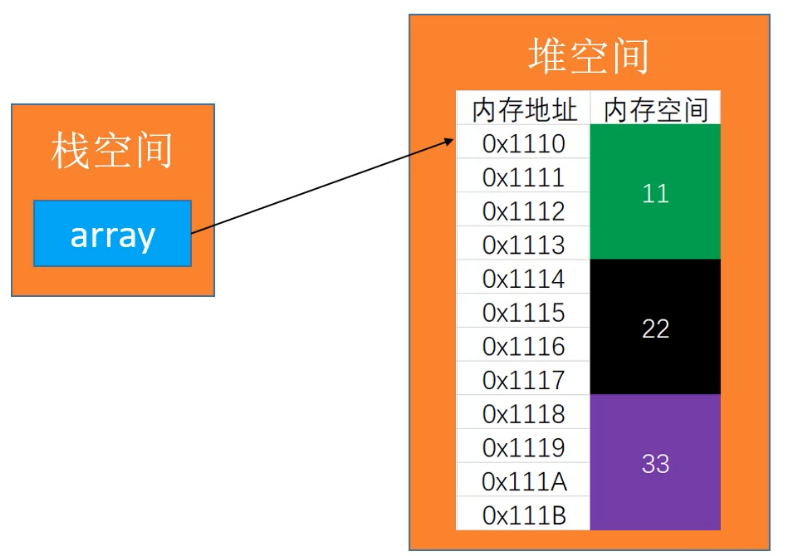• 在很多编程语言中，数组都有个致命的缺点——无法动态修改容量
• 实际开发中，我们更希望数组的容量是可以动态改变的；

## 动态数组设计

int size();                     // 元素的数量
boolean isEmpty();                 // 是否为空
boolean contains(E element);     // 是否包含某个元素
E get(int index);                 // 返回index位置对应的元素
E set(int index, E element);     // 设置index位置的元素
void add(int index, E element); // 往index位置添加元素
E remove(int index);             // 删除index位置对应的元素
int indexOf(E element);         // 查看元素的位置
void clear();                     // 清除所有元素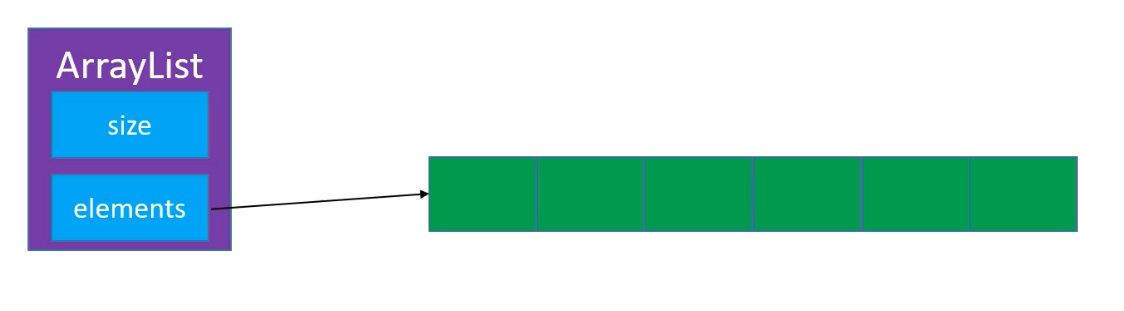public class ArrayList {
private int size;
private int[] elements;
// 默认容量为 10
private final static int DEFAULT_CAPACITY = 10;

public ArrayList(int capacity) {
// 如果小于默认容量，都为默认容量
if (capacity <= DEFAULT_CAPACITY) {
elements = new int[DEFAULT_CAPACITY];
} else {
elements = new int[capacity];
}
}
public ArrayList() {
elements = new int[DEFAULT_CAPACITY];
// 或者写成这样，调用上面重载的构造函数
// this(DEFAULT_CAPACITY);
}
}

public int size() {
return size;
}

public boolean isEmpty() {
return size == 0;
}

public int indexOf(int element) {
for (int i = 0; i < size; i++) {
if (elements[i] == element) return i;
}
return -1;
}

public boolean contains(int element) {
return indexOf(element) != -1;
}

public int get(int index) {
if (index < 0 || index >= size) {
throw new ArrayIndexOutOfBoundsException(index: " + index + ",and size: " + size);
} else {
return elements[index];
}
}
public int set(int index, int element) {
if (index < 0 || index > size) {
throw new ArrayIndexOutOfBoundsException("index: " + index + ",and size: " + size);
} else {
int old = elements[index];
elements[index] = element;
return old;
}
}
public void clear() {
// 清除数组时，只需将容量设置为 0 ，那么外层的操作都会被拦截
size = 0;
}                                                                       

private final static int ELEMENT_NOT_FOUND = -1;

## 末尾添加元素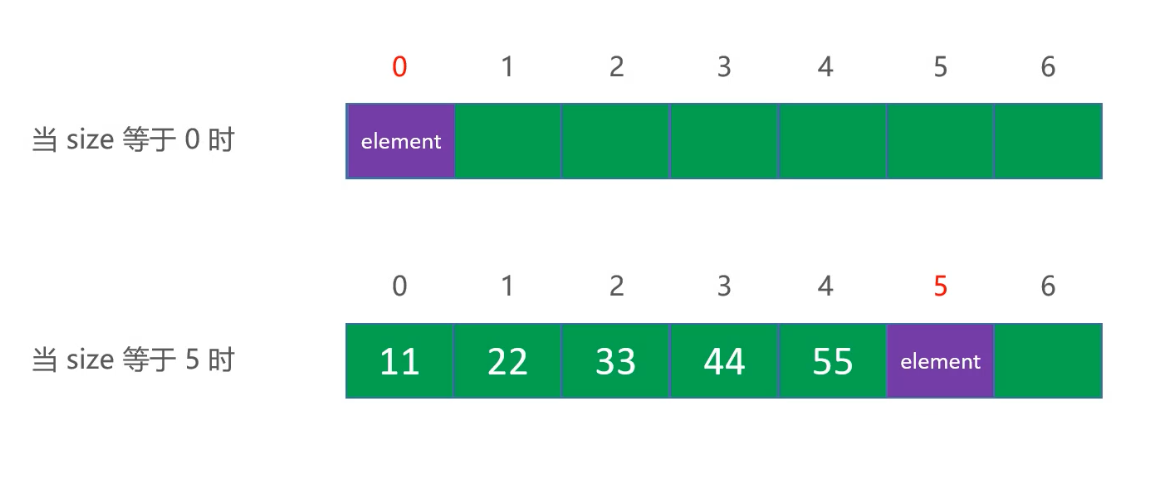public void add(int element) {
// 若容量不够，需要扩容
elements[size] = element;
size++;
}

## 打印数组

@Override
public String toString() {
StringBuilder sBuilder = new StringBuilder();
sBuilder.append("size=" + size + ", [");
for (int i = 0; i < size; i++) {
if (i != 0) {
sBuilder.append(", ");
}
sBuilder.append(elements[i]);
}
sBuilder.append(']');
return sBuilder.toString();
}

## 删除元素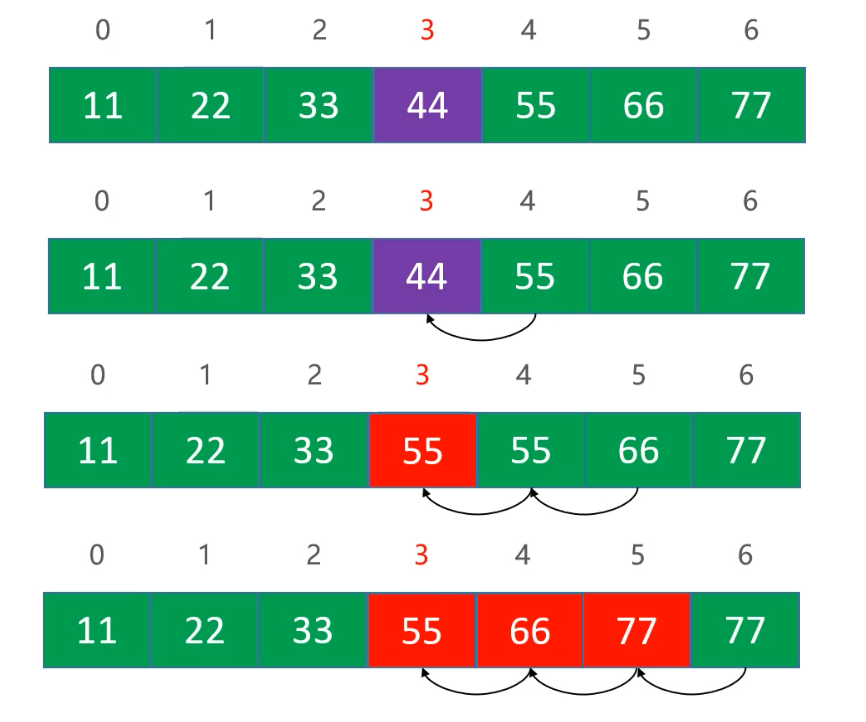public int remove(int index) {
rangeCheck(index);
int old = elements[index];
for (int i = index + 1; i < size; i++) {
elements[i - 1] = elements[i];
}
size--;
return old;
}

rangeCheck(index);其实就是对 index 合法性检查的一个封装：

private void rangeCheck(int index) {
if (index < 0 || index >= size) {
throw new IllegalArgumentException("index: " + index + ",and size: " + size);
}
}

## 指定序列添加元素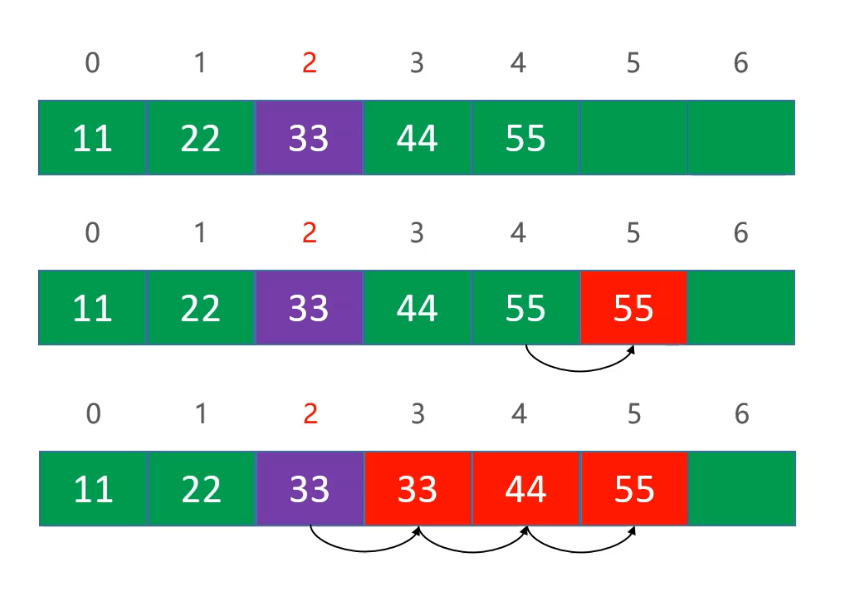public void add(int index, int element) {
for (int i = size; i > index; i--) {
elements[i] = elements[i - 1];
}
elements[index] = element;
size++;
}

private void rangeCheckForAdd(int index) {
// 这里 index == size 时则是在数组末尾添加
if (index < 0 || index > size) {
throw new IllegalArgumentException("index: " + index + ",and size: " + size);
}
}

## 接口测试

ArrayList arrayList = new ArrayList(8);
arrayList.set(0, 2);
if (arrayList.get(0) != 2) {
throw new IllegalArgumentException("测试未通过");
}


public class Asserts {
public static void test(boolean value) {
try {
if (!value) throw new Exception("测试未通过");
} catch (Exception e) {
e.printStackTrace();
}
}
}

Assert.test(arrayList.get(0)==2);

## 如何扩容

• 什么情况下需要扩容？
• 哪些接口需要扩容？

add(int index, int element)add(int element) 两个方法需要。

public void add(int element) {
}

private void ensureCapacity(int capacity) {
// 若需要满足的容量小于等于数组的值，无需扩容
if (capacity <= elements.length)
return;
// 注意运算符优先级问题，左移一位为除以二，扩容 1.5 倍
int newCapacity = capacity + (capacity >> 1);
int[] newElements = new int[newCapacity];

for (int i = 0; i < size; i++) {
newElements[i] = elements[i];
}
elements = newElements;
}

public void add(int index, int element) {
// 添加一个元素，传入需要的容量 size + 1
ensureCapacity(size + 1);
// ...
}

## 泛型支持

public class ArrayList<E> {
// ...
}

// ArrayList 初始化时，申请对象数组
elements = new E[capacity];
// Cannot create a generic array of E

@SuppressWarnings("unchecked")
public ArrayList(int capacity) {
if (capacity <= 0) {
elements = (E[]) new Object[DEFAULT_CAPACITY];
} else {
elements = (E[]) new Object[capacity];
}
}

### 对象数组 & 内存管理细节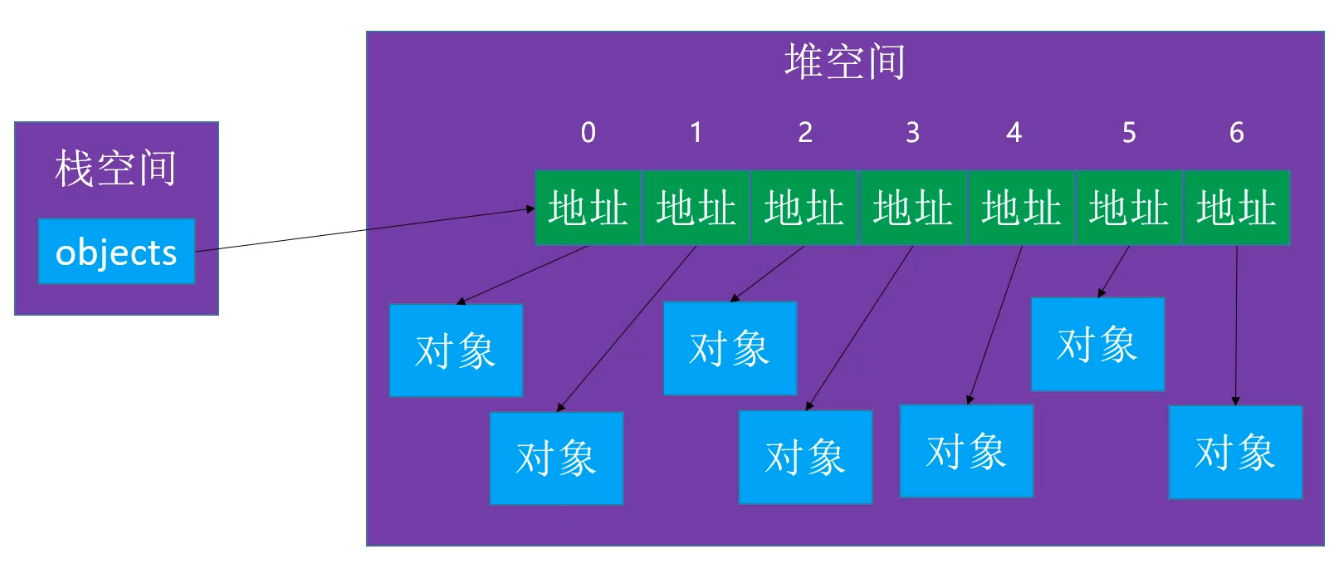public void clear() {
for (int i = 0; i < size; i++) {
elements[i] = null;
}
size = 0;
}

// 测试对象
public class Person {
private String name;
private int age;

public Person(String name, int age) {
super();
this.name = name;
this.age = age;
}

@Override
public String toString() {
return "Person [name=" + name + ", age=" + age + "]";
}

@Override
protected void finalize() throws Throwable {
super.finalize();
System.out.println("Person finalize");
}
}

// 测试用例
ArrayList<Person> arrayList = new ArrayList<>();

System.out.println(arrayList.toString());
arrayList.clear();
// 提醒 JVM 垃圾回收
System.gc();

public E remove(int index) {
rangeCheck(index);
E old = elements[index];
for (int i = index + 1; i < size; i++) {
elements[i - 1] = elements[i];
}
elements[--size] = null;
return old;
}

### equals & null 的处理

@Override
public boolean equals(Object obj) {
Person person = (Person) obj;
return person.age == age;
}

public int indexOf(E element) {
if (element == null) {
for (int i = 0; i < size; i++) {
if (elements[i] == null)
return i;
}
} else {
for (int i = 0; i < size; i++) {
if (element.equals(elements[i]))
return i;
}
}
return ELEMENT_NOT_FOUND;
}

## 动态缩容

private void trimCapacity() {
int newCapacity = elements.length >> 1;
if (size <= newCapacity && size > DEFAULT_CAPACITY) {
@SuppressWarnings("unchecked")
E[] newElements = (E[]) new Object[newCapacity];
System.out.println(elements.length + ",缩容为： " + newElements.length);
for (int i = 0; i < size; i++) {
newElements[i] = elements[i];
}
elements = newElements;
}
}

目录